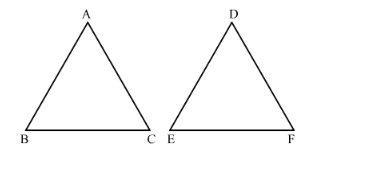# If in two triangles ABC and DEF, ABDE=BCFE=CAFD, then

Question:

If in two triangles ABC and DEF, ABDE=BCFE=CAFD, then

(a) ∆FDE ∼ ∆CAB
(b) ∆FDE ∼ ∆ABC
(c) ∆CBA ∼ ∆FDE
(d) ∆BCA ∼ ∆FDE

Solution:

We know that if two triangles are similar if their corresponding sides are proportional.It is given that $\triangle \mathrm{ABC}$ and $\triangle \mathrm{DEF}$ are two triangles such that $\frac{\mathrm{AB}}{\mathrm{DE}}=\frac{\mathrm{BC}}{\mathrm{EF}}=\frac{\mathrm{CA}}{\mathrm{FD}}$.

∠A=∠D∠B=∠E∠C=∠F

$\therefore \triangle \mathrm{CAB} \sim \triangle \mathrm{FDE}$

Hence the correct answer is $(a)$.